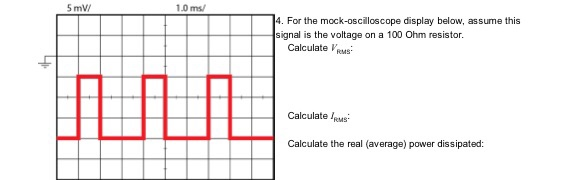### Create an Account

Home / Questions / 5 mV 1.0 ms/ 4. For the mock-oscilloscope display below, assume this signal is the voltage on a 100

# 5 mV 1.0 ms/ 4. For the mock-oscilloscope display below, assume this signal is the voltage on a 1005 mV 1.0 ms/ 4. For the mock-oscilloscope display below, assume this signal is the voltage on a 100 Ohm resistor Calculate Veus Calculate Calculate the real (average) power dissipated:

Apr 06 2020 View more View LessSubscribe To Get Solution